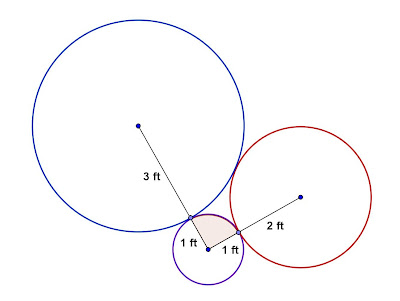## Monday, December 31, 2012

### Three Tangent Circles

Category: Plane Geometry

"Published in Suisun City, California, USA"

Three circles with radii 1, 2, and 3 ft. are externally tangent to one another, as shown in the figure. Find the area of the sector of the circle of radius 1 ft. that is cut off by the line segments joining the center of that circle to the centers of the other two circles.Photo by Math Principles in Everyday Life

Solution:

If you connect the centers of three circles by line segments, the figure is a triangle as shown belowPhoto by Math Principles in Everyday Life

The length of the sides of a triangle is calculated by the sum of the two radii that are tangent to each other. We notice that the sides of a triangle are the sides of a right triangle. Why? We can prove this by Pythagorean Theorem as follows

Therefore, a triangle is a right triangle. The right angle is located at the opposite side of hypotenuse which is 5 ft. The angle of the sector for the circle with a radius of 1 ft. is a right angle also or 90º.

Note: If a triangle is not a right triangle, then we have to use the Cosine Law in order to get the angle which is located at the opposite side of hypotenuse.

Since we know the angle of a sector of a circle with a radius of 1 ft., then we can calculate the area of the sector as follows

where: r = radius of a sector

θ = angle of a sector in radians (a unit less angle)

The value of θ in radians is calculated as follows

Therefore, the area of a sector is